Test: Block Diagrams

# Test: Block Diagrams

Test Description

## 10 Questions MCQ Test GATE Electrical Engineering (EE) 2023 Mock Test Series | Test: Block Diagrams

Test: Block Diagrams for Electronics and Communication Engineering (ECE) 2023 is part of GATE Electrical Engineering (EE) 2023 Mock Test Series preparation. The Test: Block Diagrams questions and answers have been prepared according to the Electronics and Communication Engineering (ECE) exam syllabus.The Test: Block Diagrams MCQs are made for Electronics and Communication Engineering (ECE) 2023 Exam. Find important definitions, questions, notes, meanings, examples, exercises, MCQs and online tests for Test: Block Diagrams below.
Solutions of Test: Block Diagrams questions in English are available as part of our GATE Electrical Engineering (EE) 2023 Mock Test Series for Electronics and Communication Engineering (ECE) & Test: Block Diagrams solutions in Hindi for GATE Electrical Engineering (EE) 2023 Mock Test Series course. Download more important topics, notes, lectures and mock test series for Electronics and Communication Engineering (ECE) Exam by signing up for free. Attempt Test: Block Diagrams | 10 questions in 30 minutes | Mock test for Electronics and Communication Engineering (ECE) preparation | Free important questions MCQ to study GATE Electrical Engineering (EE) 2023 Mock Test Series for Electronics and Communication Engineering (ECE) Exam | Download free PDF with solutions
 1 Crore+ students have signed up on EduRev. Have you?
Test: Block Diagrams - Question 1

### The transfer function of the control system represented by the block diagram shown below is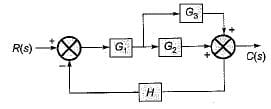Detailed Solution for Test: Block Diagrams - Question 1

On combining the blocks G2 and G3 which are in parallel, the given block diagram will be reduced as shown below.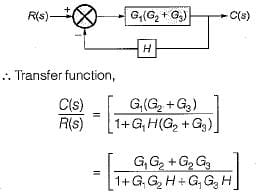Test: Block Diagrams - Question 2

### Which one of the following block diagrams in options given is equivalent to the below shown block diagram?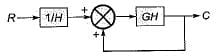Detailed Solution for Test: Block Diagrams - Question 2

The T.F. of the given block diagram can be reduced to: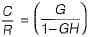Its block diagram is shown below, where a closed loop feedback system is transformed into an unity feedback system and vice-versa,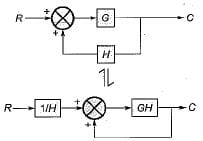Test: Block Diagrams - Question 3

### Consider the following statements: 1. Transfer function is an expression which relates output to input in s-domain. 2. Transfer function gives information about the internal structure of the system. 3. A system can be represented by the block diagram if the transfer function of the system is known. 4. Block diagram is the flow of system variables from one block to another block represented by a single line. Q. Which of the above statements is/are true?

Detailed Solution for Test: Block Diagrams - Question 3

Transfer function does not gives information about the internal structure of the system. Hence, staement-2 is false.

Test: Block Diagrams - Question 4

The ratio C(s)/R(s) for the system shown in figure below is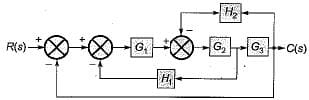Detailed Solution for Test: Block Diagrams - Question 4

On shifting the take-off point beyond the block G3 and combining blocks G2 and G3, the given block diagram is reduced as shown below.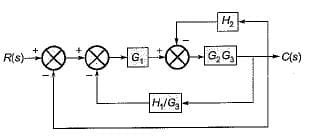The block diagram is further reduced as shown below.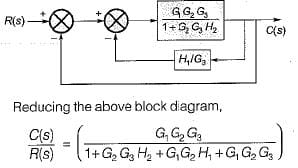Test: Block Diagrams - Question 5

The transfer function C(s)/R(s) for the system described by the block diagram shown below is given by: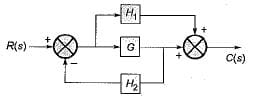Detailed Solution for Test: Block Diagrams - Question 5

On shifting the take-off point beyond block G, we have the reduced block diagram as shown below: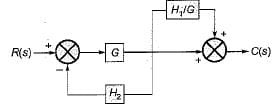On further reducing the above block diagram, we get the block diagram as shown below.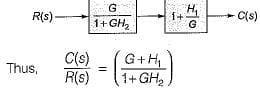Test: Block Diagrams - Question 6

The transfer matrix for the multi input-multi output (MIMO) system represented by the block diagram shown below is given by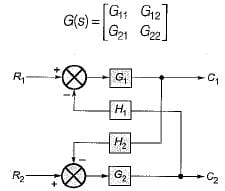Match List-I with List-II and select the correct answer using the codes given below the lists: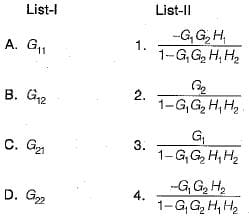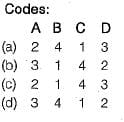Detailed Solution for Test: Block Diagrams - Question 6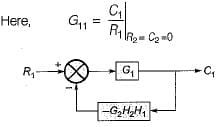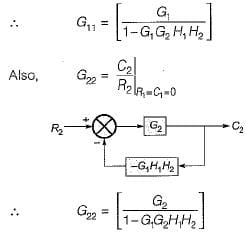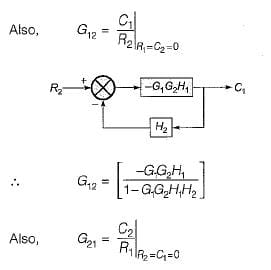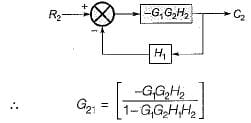Test: Block Diagrams - Question 7

Consider the three block diagrams A, B and C shown below.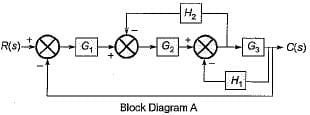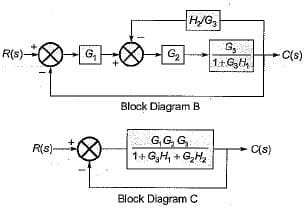Q. Which one of the following statements is correct in respect of the above block diagrams?

Detailed Solution for Test: Block Diagrams - Question 7

Shifting the take-off point after block G3 in block diagram-A, the reduced block diagram will be as shown below.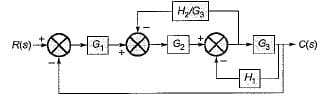Reducing further, the block diagram is reduced as shown below.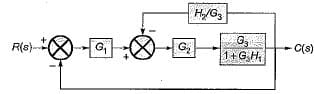Thus, the block diagrams A and B are equivalent to each other.
On further reducing the block diagram-B, we get the block diagram-C. Hence, all the three block diagrams A, B and C are equivalent to each other,

Test: Block Diagrams - Question 8

The poles of the transfer function C(s)/R(s) of the system represented by the block diagram shown below are located at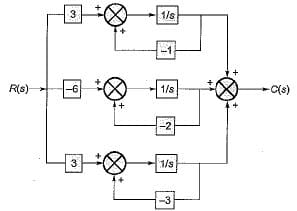Detailed Solution for Test: Block Diagrams - Question 8

The given block diagram can be reduced as shown below.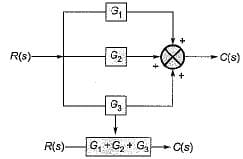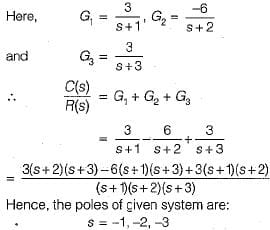Test: Block Diagrams - Question 9

The transfer function C(s) / R(s) for the block diagram shown below is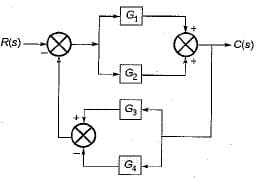Detailed Solution for Test: Block Diagrams - Question 9

Combining the blocks in parallel, the block diagram is reduced as shown below.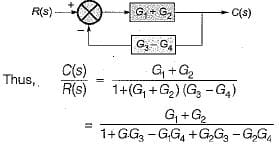Test: Block Diagrams - Question 10

The value of for the system described by the block diagram shown in figure below Is.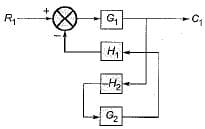Detailed Solution for Test: Block Diagrams - Question 10

The given block diagram can be reduced as shown below.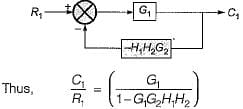## GATE Electrical Engineering (EE) 2023 Mock Test Series

22 docs|274 tests
Information about Test: Block Diagrams Page
In this test you can find the Exam questions for Test: Block Diagrams solved & explained in the simplest way possible. Besides giving Questions and answers for Test: Block Diagrams, EduRev gives you an ample number of Online tests for practice

## GATE Electrical Engineering (EE) 2023 Mock Test Series

22 docs|274 tests(Scan QR code)